﻿ Statistical Analysis and Evaluation of Drinking Water Consumption Needs in Dakar from 2016 to 2025Publications are Open
Access in this journal
Article Versions
Export Article
• Normal Style
• MLA Style
• APA Style
• Chicago Style
Original Article
Open Access Peer-reviewed

### Statistical Analysis and Evaluation of Drinking Water Consumption Needs in Dakar from 2016 to 2025

Ndiouga CAMARA , Ibrahima MBAYE, Déthié SARR, Séni TAMBA
American Journal of Water Resources. 2021, 9(1), 1-7. DOI: 10.12691/ajwr-9-1-1
Received November 20, 2020; Revised December 24, 2020; Accepted January 07, 2021

### Abstract

In Dakar, a considerable deficit is observed in the distribution of drinking water. The demand for drinking water is increasing day by day and the available resources are insufficient. The objective of this article is first to assess the drinking water consumption needs of the Dakar region by performing a statistical analysis of the data. Statistical analysis of the data made it possible to assess the state of coverage of drinking water consumption needs. In addition, this statistical analysis will make it possible to make a comparison between the evolution of drinking water consumption needs and the current available resources. The results obtained show that after interpolations of the data by different polynomials (interpolation of degree 1, interpolation of degree 5, and interpolation of degree 10), the best interpolation in order to have an optimal projection of the evolution of drinking water consumption as a function of the population is the interpolation polynomial of degree 10. This equation called the Dakar equation which gives the consumption as a function of the population is as follows: Cons = 8,959.102Pop2 – 5,2592.108Pop + 1,382.1014.

### 1. Introduction

Statistics will make it possible to analyze the data, in order to comment on or interpret the facts to which these data relate.

These are the data relating to the population and the consumption of drinking water.

These data will be presented in an appropriate manner to enable meaningful results to be determined and appropriate comments to be made.

The calculation of drinking water needs relates to the estimate of the workforce to be served, that is to say the population affected by the network and therefore the determination of any water needs.

To determine the current and future needs for drinking water, we must determine the needs for the resident population, the needs for public and social facilities without forgetting the needs for individuals.

We would therefore like to point out that the objective of the drinking water supply system is to meet as much as possible the water needs of the area concerned by the project for a well-defined period which is estimated as the lifespan of the network.

The water needs correspond to the supply that the operator will have to make available to meet user demand. Drinking water requirements are determined at each stage of the system by taking into account the users' drinking water demand, their behavior and the yields of the installations concerned 1.

Short, medium and long term water demand is calculated on the basis of average consumption or demand. It is made on the basis of specific average daily consumption and the results of surveys of institutional and socio-economic users.

Specific consumption and service rates were defined from consumption analyzes from the statistical file of SEN EAU (formerly SDE) and in conformity as far as possible with the Millennium Development Goals 2, 3.

### 2. Materials and Methods

2.1. Data Acquisition

To assess the evolution of consumption, we develop graphs (curves) which give the consumption values in m3 / day according to the years (from 1997 to 2025). We will also determine the mean of the values and the standard deviation 4.

The latter allows us to learn about the dispersion of values around the mean.

Data analysis is necessary and consists of describing and exploring the data to derive some laws or predictive models.

Thus, we have gathered the data relating to population and consumption from 1997 to 2018 in Table 1.

This table shows the population in number of inhabitants for each year from 1997 to 2018; but also the annual consumption in m3 from 1997 to 2018.

This table allows us to see how the population and consumption of drinking water in Dakar evolved during this period.

We were able to obtain 22 years of drinking water consumption data at the SDE level.

The latter did not want to provide data prior to 1996; date on which his affermage contract with the state of Senegal began.

All the data on the overall consumption of the Dakar region as well as data on the population from 1997 to 2018 are shown in Table 1.

From Table 1, we have established, using Matlab software, the point clouds of different polynomials (interpolation of degree 1, interpolation of degree 5, and interpolation of degree 10).

2.2. Data Processing and Analysis (Numerical Statistics)

The Dakar region is made up of four (4) departments (Figure 1).

The analysis and processing of digital data was facilitated by the Matlab software which allowed us to draw figures and curves; which will make it possible to derive the equations for the evolution of drinking water consumption in the Dakar region.

• Figure 1. Departmental division of the Dakar region

The assessment of drinking water needs was made on the basis of the calculation assumptions set out in the Table 2.

For the situation of water supply sources, the Senegalaise des Eaux (SDE), linked to the State of Senegal by a contract, provides drinking water supply to populations in urban and peri-urban areas. It has Eleven (11) Regional Directorates and the Dakar region has three (03) in which the 14 centers of this study are attached as shown in Table 3 5.

### 3. Results and Discussions

From Table 1, we established, using Matlab software, the point clouds and the regression line in Figure 2, which show how the population and water consumption in Dakar evolved from 1997 to 2018.

• Figure 2. Curve of the consumption (m3) of drinking water compared to the population from 1997 to 2018 from the polynomial of degree 1 interpolation

Figure 2 shows a scatter plot of drinking water consumption versus population as well as the regression line.

The following expression allowed us to calculate the correlation coefficient between the population and the consumption of drinking water.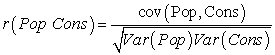Thanks to this expression, we found a correlation coefficient r (Pop, Cons) = 0.9559.

This coefficient r (Pop, Cons) = 0.9559 is close to 1. So we have a good correlation. This strong correlation shows that population and consumption are linearly related.

Therefore, a functional relationship can be established between population and consumption. That is, find a function f such that Y = f (X).

The idea is to find a polynomial fit.

3.1. Approximation by an interpolation polynomial of degree 1.

We are trying to approach the point cloud on a straight line.

So, we can use a linear fit such that: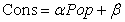(1)

Thus, thanks to the programming done with the Matlab software, we have the following values of α and β: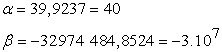In addition, we have output for the population as well as for the consumption of drinking water, the values of the mean, standard deviation and variance.

Standard deviation is a measure of the dispersion of values in a statistical sample or probability distribution. It is defined as the square root of the variance or, equivalently, as the root mean square of the deviations from the mean.

If the standard deviation is greater than 0.5 mean, we can therefore consider that the variations are strong.

These values (means, standard deviations, variances) are recorded in Table 4.

Table 4 gives high values of the standard deviation, this means that the values are scattered around the mean (heterogeneous series).

So we can conclude that the values are widely distributed.

Also, if we replace α and β with their values, the linear fit 1 becomes:(2)

From this relationship, we have established a projection of consumption as a function of the population until the year 2025 (Table 5).

3.2. Approximation by an Interpolation Polynomial of Degree 5.

Next, we have established the point clouds given by the interpolation polynomial of degree 5 in Figure 3.

Figure 3 shows how water consumption in Dakar has changed in relation to the population from 1997 to 2018 according to the polynomial interpolation of degree 5.

• Figure 3. Curve of the consumption (m3) of drinking water compared to the population from 1997 to 2018 from the interpolation polynomial of degree 5

We used a polynomial interpolation of degree 5 which gives:(3)

We have the following values of α’, β’, γ’, θ’, δ’ and c’: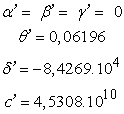Therefore, if we replace α’, β’, γ’, θ’, δ’and c with their values, the polynomial interpolation of degree 5 becomes: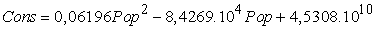(4)

In addition, in 2005, the stoppage of drilling in Grand Medina due to a breakdown and those in Thiaroye due to public health problems (contamination of the water table) made production constant while demand increased. This explains the plateau at the level of the curve of the interpolation polynomial of degree 5.

From equation 4, we have established Table 6 which gives a projection of drinking water consumption compared to the population from the year 2019 to the year 2025.

3.3. Approximation by an Interpolation Polynomial of Degree 10

Finally, we have established the point clouds given by the interpolation polynomial of degree 10 in Figure 4.

Thus, Figure 4 shows how water consumption in Dakar has evolved compared to the population from 1997 to 2018 according to the polynomial interpolation of degree 10.

Thus, the results of polynomial interpolation of degree 10 give: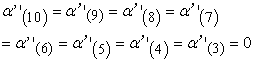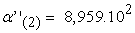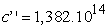Therefore, if we replace the coefficients with their values, the polynomial interpolation of degree 10 becomes: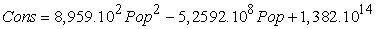(5)

From this relationship, we have established Table 7 which gives a projection of drinking water consumption compared to the population from the year 2019 to the year 2025.

• Figure 4. Curve of the consumption (m3) of drinking water compared to the population from 1997 to 2018 from the interpolation polynomial of degree 10
3.4. Interpretation of the Errors of the Different Interpolations

The results of the calculations of the different interpolations (degree 1, degree 5, degree 10) gave the margins of error for the different polynomials studied.

These margins of error are recorded in Table 8.

From Table 8, the trend curves of Figure 5 are established. These curves will make it possible to interpret the reliability of the polynomial interpolations but also to designate the optimal polynomial in order to establish a better projection of the evolution of drinking water consumption in the Dakar Region.

• Figure 5. Trend curves of the margins of error of the different polynomials studied

Figure 5 shows that the polynomial of degree 10 has very small margins of error compared to other polynomials. For some years (2020, 2024 and 2025), the margins of error are even negligible.

Therefore, the best interpolation in order to have an optimal projection of the evolution of drinking water consumption as a function of the population is the polynomial of degree 10.

This polynomial of degree 10 is given by equation 5.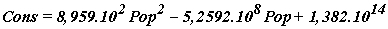### 4. Conclusion

We have tried several polynomial interpolations (degree 1, degree 5 and degree 10).

According to the results obtained, the Dakar equation, the best interpolation in order to have an optimal projection of the evolution of drinking water consumption as a function of the population, is given by the polynomial of degree 10.

This polynomial of degree 10 is given by the following equation 5:In addition, the infrastructures built constitute, by their number and their very varied functions, not only a real attraction, but also a demographic amplifier.

In fact, special attention must be paid to the assessment of available drinking water resources in relation to future needs.

### References

  C. Sophie and L. Jean, “Drinking water service: From the logic of supply to control of demand,” Jwarp, 1996. In article  SDE, “ANNUAL STATISTICAL SHEET,” 2009. In article  PEPAM, “ASSUMPTIONS FOR THE MILLENNIUM DEVELOPMENT GOALS,” 2015. In article  B. Igor and G. Didier, “Tool to help diagnose the drinking water network for the city of chisinau by spatial and temporal analysis of hydraulic dysfunctions,” jwarp, 2004. In article  SONES, "Hydrochemical study of the quaternary sand table in the Dakar area," 2012. In article  Béture-Sétane., “Strengthening of Dakar's water supply,” 1988. In article  G. C. F. A. FAYE S, Modeling of the hydrodynamic functioning of the aquifer of the northern coast of Senegal. Simulation of additional withdrawals to reduce the drinking water distribution deficit in the Dakar region. BRGM Hydrogeology N01-pp. 13-22, 1998. In article  C. MERLIN, Drinking water supply project for towns in the central zone and on the coast. Dakar, 386p. July 2010. In article  N. BATHILY, map of the departments of the Dakar region, June 2017. In article  S. Faye, “Hydrodynamic modeling of the water tables of the north coast between Kayar and Saint-Louis. Impact of future withdrawals envisaged within the framework of the water supply of Dakar and its surroundings,” Dakar, 1995. In article  SDE, “Study to establish master plans and a database of urban hydraulics,” 2012. In article  DGPRE, “Basic data, needs-resources assessment and diagnosis of existing installations,” 2012. In article  ANSD / RGPHAE, “Resurgence of the population of Senegal,” 2013. In article  C. M. S. SONES, Mobilization of alternative water resources for irrigation in the Dakar region. 79 p. 2007. In article  C. MERLIN, “Water Supply Project for Cities in the Center and Littoral Zone. Dakar,” (July 2010). In article  SONES, “Dakar Special Drinking Water Supply Program,” 2018. In article  COWI-POLYCONSULT, “Control of production facilities in Dakar and Banlieu,” 2009. In article  SETICO / CONCEPT, “Possible variables for mobilizing water resources,” 2013. In article  SDE / SONES, “Establishment of the master plan, phase 2 report,” 2013. In article  ANSD, “Report on the census of the population of the Dakar region,” 2015. In article

Published with license by Science and Education Publishing, Copyright © 2021 Ndiouga CAMARA, Ibrahima MBAYE, Déthié SARR and Séni TAMBAThis work is licensed under a Creative Commons Attribution 4.0 International License. To view a copy of this license, visit http://creativecommons.org/licenses/by/4.0/